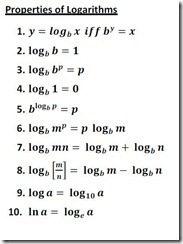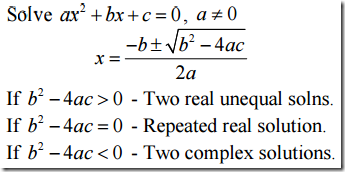## Tuesday, April 26, 2016

### Logarithms–Important Laws & Formulas

Q) Why log 1=0?
The important thing to remember is the log represents the exponent.  In the case of common logs, the base is always base 10.  Now lets see some examples which clears your doubt:
1) log 100 = 2 because 102 = 100.
2) log 1000 = 3 because 103 = 1000.
3) log 1 = 0 because 100 = 1.
4)  log .1 = -1 because 10-1 = .1
5)  log .01 = -2 because 10-2 = .01Q) How to change base in logarithms?
We can change any base to a different base any time we want. The most used bases are obviously base 10 and base e.
Change of base formula:  Logb x = Loga x/Loga b

## Sunday, April 24, 2016

Given the General Equation - ax2 + bx + c = 0, will have 2 roots, as the highest power in the equation is 2.
Those 2 roots are given by the equation  x = -b ± √(b2 -4ac) / 2a

Further, the questions in SSC wont ask you directly to find the roots. Instead he may ask:
a)  Sum of Roots of a quadratic equation = -b/a
b) Product of the roots of a quadratic equation= c/a